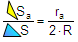# Geometry Problem 83. Area of a Excircle Contact Triangle, Exradius, Circumradius. High School, College, Math Education

 Given a triangle ABC of area S, the excircle of center D, the exradius ra, and the circumradius R. If Sa is the area of the contact triangle EFG, from the tangent points of the excircle and triangle ABC, prove that:.HINTS: 1. See Problems 80, 81, 82. CONTACT TRIANGLE: The contact triangle of a triangle ABC (figure above), also called the intouch triangle, is the triangle DEF formed by the points of tangency of the incircle of triangle ABC with triangle ABC.   AREA OF A TRIANGLE: Semiperimeter and Exradius FormulaHome | Search | Geometry | Problems | All Problems | Open Problems | Visual Index | Art | 81-90 | Circle Tangent Line | Email | View or post a solution | by Antonio Gutierrez